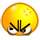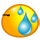# [Signal processing with Python] Bài 1 (tt): WAV file

Discussion in 'Python' started by Ngô Văn Tuân, May 17, 2019.

1. ### Ngô Văn TuânThành Viên PIF

4. Thực hành:

Lưu ý: Nếu bạn chưa hiểu được bài giải thì cứ copy vào chạy trước đã, giải thích code sẽ được trình bày ở những bài sau.

a. Tạo sóng sin với tần số 1000Hz và lưu dưới định dạng wav.

import numpy as np
import wave
import struct
import matplotlib.pyplot as plt

# Create sine wave
sampling_rate = 44100
frequency = 1000
num_samples = 88200
sine_wave = []
for x in range(num_samples):

sine_wave.append(np.sin(2 * np.pi * frequency * x * 1/sampling_rate))
# Save sine wave as Wav file
amplitude = 8000
file = "test.wav"
wav_file=wave.open(file, 'w')
nframes=num_samples
comptype="NONE"
compname="not compressed"
nchannels=1
sampwidth=2
wav_file.setparams((nchannels, sampwidth, int(sampling_rate), nframes, comptype, compname))
for s in sine_wave:

wav_file.writeframes(struct.pack('h', int(s*amplitude)))
wav_file.close()

# Plot sine wave
plt.plot(sine_wave[:300])
plt.show()
Hãy thay đổi frequency để xem tai bạn có thể nghe được âm thanh có tần số lớn nhất là bao nhiêu.
b. Mở file "cartoon008.wav" và add sine noise vừa tạo vào file âm thanh đó.

import numpy as np
import struct
import wave

infile = "cartoon008.wav"
wav_file = wave.open(infile, 'r')
length = wav_file.getnframes()
data = list(struct.unpack("<" + str(length) + "h", wave_data))
wav_file.close()

num_samples = length
frequency = 15000
amplitude = 8000
sampling_rate = 44100
for x in range(num_samples):

noise = np.sin(2 * np.pi * frequency * x/sampling_rate)
data[x] = data[x] + noise*amplitude
file = "test.wav"
wav_file=wave.open(file, 'w')
nframes=num_samples
comptype="NONE"
compname="not compressed"
nchannels=1
sampwidth=2
wav_file.setparams((nchannels, sampwidth, int(sampling_rate), nframes, comptype, compname))
for s in data:

wav_file.writeframes(struct.pack('h', int(s)))
wav_file.close()
Hãy thay đổi biên độ và tần số của sóng sin để nghe được âm thanh nhiễu nhé.
Hết bài 1.

Last edited: May 17, 2019